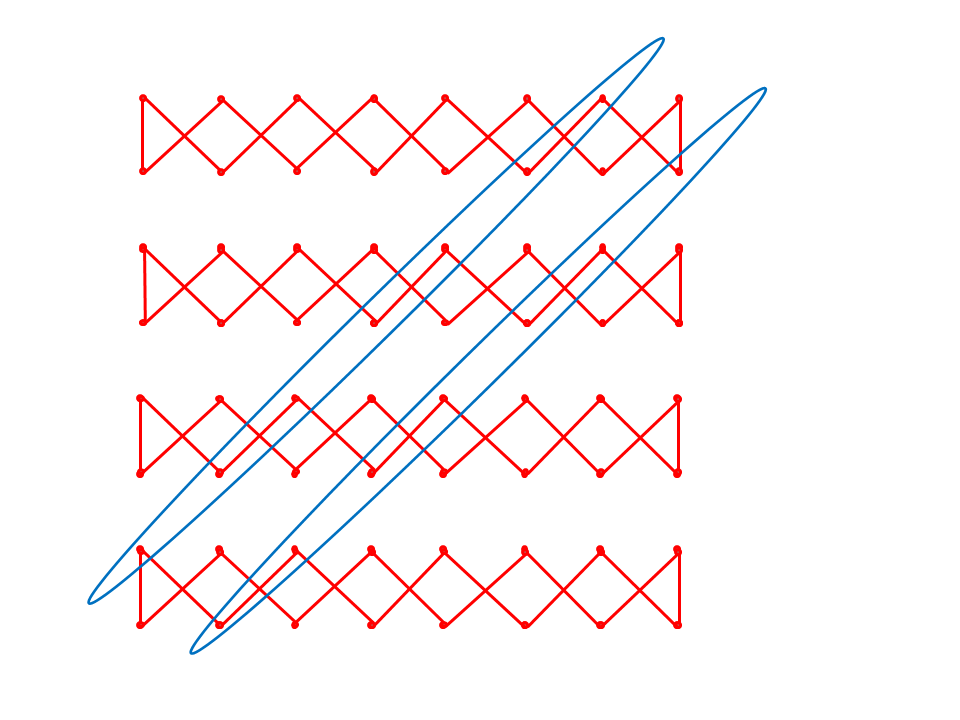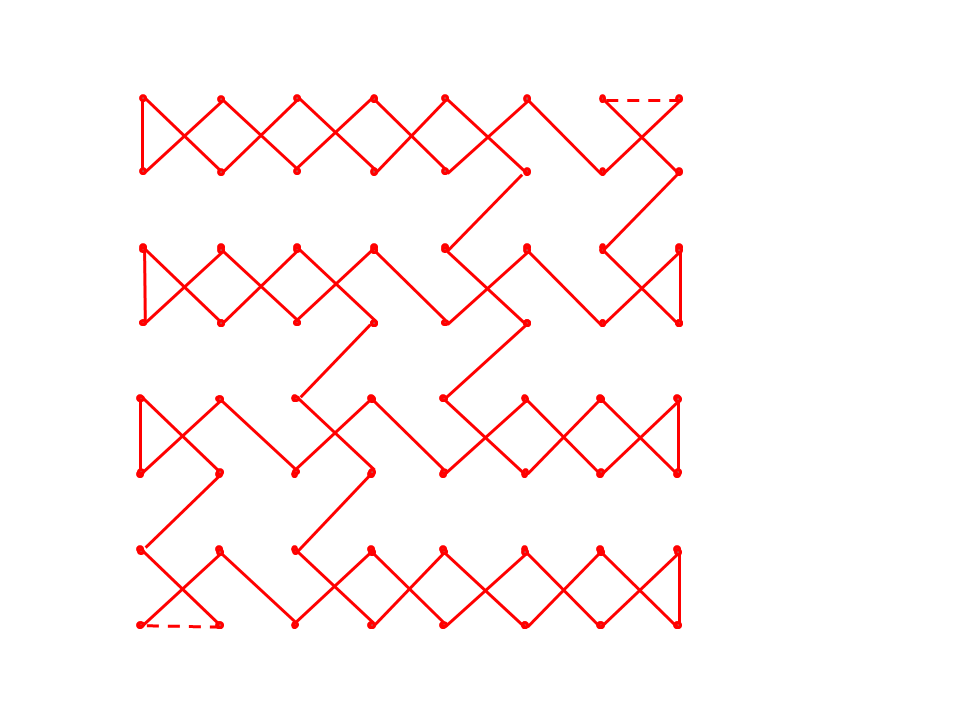## October 2013 solution

The maximal length is 120, and the solution, up to mirroring, reflection, tour directionality and starting position, is either
```0062026004585556
6301610359055754
4345414706495351
4442464048075052
2018391608141012
1921173815091311
2225372735293331
2423263628343032
```
or
```0062596057515553
6301615850565254
0245044706490810
4403460548071109
4143391637143512
4240173815361334
2220241826293331
2123192528273032
```
In a king's tour, every move is either axis-parallel or diagonal. Diagonal moves contribute 2 to the tour length and axis-parallel ones only 1. Because the total number of moves is a constant (64), maximizing the tour length is minimizing the number of axis-parallel moves. The total tour length is 128 minus the number of axis-parallel moves.

In the solutions given above there are only 8 axis-parallel moves. We now prove that it is impossible to solve with less.

To see this, consider a corner of the board. The king can move to it or from it diagonally, but not both together. For this reason, each corner contributes at least one, and possibly two axis-parallel moves. Let us assume that it contributed only one, then there is a diagonal motion to/from the corner. Let us take the example of corner "a1". There must be king move that connects "a1" to "b2". Now, from "b2", the king may go to "a3" or it may go to "c1" (or neither), but it cannot go to both. Therefore, one of these possible moves is not taken. Consider squares "a3" and "c1". Each of these has only two diagonals connecting to it. Because at least one of these diagonals is known not to have been taken, the king must have moved to/from one of these squares using an axis-parallel move. (And, to be strict, I will add that both "a3" and "c1" are too far away from "a1" for this to have been the same axis-parallel move that was already counted for the corner itself.) In total, within the quadrant of the investigated corner, there must have been at least two axis-parallel moves.

Repeating the same analysis for all 4 quadrants, we see that there must have been, in total, at least 4*2=8 axis-parallel moves.

A similar, and only slightly longer case-by-case analysis (not shown here) can be used to verify that the solutions shown above are the only ones.

But what about the general case of a (2k)*(2k) board?

First, let us describe the king path using a different notation. Consider the chessboard in Image 1.Image 1

Within each square we have drawn a dot in red. Throughout the remainder of this explanation, each square will be represented by its red dot. A line between two dots indicates that the king has moved between the two squares in a single move.

Now, consider Image 2.Image 2

This image depicts a covering of the chessboard by several cycles. The same pattern can be extended to any (2k)*(2k) board. It uses only 2k axis-parallel moves. (However, of course, we are looking for a covering by a single cycle, not by multiple cycles.)

One interesting fact regarding the construction of Image 2 is that if one is to look at any diagonal of the chessboard, the paths taken by the king are such that one interlaces between diagonal steps taken by the king along the diagonal and steps not taken by the king along the diagonal. If the king moved diagonally along one segment, it will not continue straight along the same diagonal, and if it did not move diagonally along a segment, it will surely do so in the next segment.

This pattern is exemplified in Image 3, where two diagonals are highlighted. (They are encircled by ellipses.) These are two diagonals directly adjacent to a main diagonal of the chessboard.

(More simply stated, this pattern can be described as follows: the king makes a 90 degree turn at every internal board position. Todd Will, having checked all solutions for various even board sizes, tells me that this is a property shared by all of them. Thanks Todd! Very interesting.)Image 3

Consider now what would happen if one were to take the edges along these two diagonals and invert them: where a king did not move before, it moves now, and where it moved before it no longer moves now. After a minor fixing of the axis-parallel moves, we get Image 4. (The new axis-parallel moves are shown as dashed lines.)Image 4

This is now a single cycle with only 2k axis-parallel moves.

At the time of this riddle's publication, I had no proof that this construction is optimal in the general case. However, Lorenz Reichel sent in the most amazingly elegant proof of this optimality. I am including his e-mail to me here, essentially verbatim. (The e-mail discusses the 8x8 case, but all its argumentation is trivially applicable also in the general case.)

He writes:

"We will show that the maximal length such a king's path can have is 120. We do this by showing that each such path must contain at least 8 straight (straight := non-diagonal) steps of the king.

We consider the standard black and white chessboard coloring. We notice that each diagonal king step connects two black or two white squares, while a straight step connects two different colored squares. A diagonal king step between two black squares we call a black step. We say that a black segment is a maximal subpath of the king, where he visits only black squares, i.e. before and after the black segment the king is on a white square. A black segment can have length zero, i.e. it consists of the visit of a single black square between whites. Note that all black segments together must cover exactly all black squares.

We will show that there must be at least four black segments. Having proven that, we are done, as before and after each black segment, i.e. at least 8 times, the king moves between different colored squares. And thus the path contains at least 8 straight steps.

To show that at least four black segments are needed to cover the black squares of a chessboard, we color the black squares as given in Image 5. We will count the number of endpoints of black segments. For a black segment of length zero, the single square it contains counts as two endpoints. In this way, each black segment has exactly two endpoints.

Image 5

Note that each black square either

• connects to two black squares by black steps,
• connects to one black square by a black step and is an endpoint of a black segment, or
• is the double endpoint of a black segment.

Thus the number of black steps leading to a black square plus the number of black segment endpoints on that square always equals two.

We count the number of black steps that connect a blue square with another square. This other square must be either red or green.

• At most 12 black steps can connect a blue with a red square, as there are 6 red squares.
• At most 4 black steps can connect a blue with a green square, as there are 4 such black steps possible on the board.

It follows that at most 16 black steps can connect a blue square with another square. Since there are 10 blue square, there are at least 2*10-16 = 4 endpoints of black segments on blue squares.

Similarly, we can show there are at least 4 endpoints of black segments on green squares.

Thus, there are at least 8 endpoints of black segments and hence 4 black segments. We are done."

My thanks, again, to Lorenz Reichel for this fantastic proof, as well as to Yaron Gvili for coming up with the riddle in the first place.